Electromagnetic Interaction

Also found in: Dictionary, Thesaurus, Medical, Wikipedia.
Related to Electromagnetic Interaction: Electromagnetic force, Electromagnetic theory

electromagnetic interaction

[i¦lek·trō·mag′ned·ik ‚int·ə′rak·shən]
(particle physics)
The interaction of elementary particles that results from the coupling of charge to the electromagnetic field.

Electromagnetic Interaction

one of the four known fundamental interactions, the other three being the gravitational, weak, and strong interactions. The electromagnetic interaction is characterized by the participation of an electromagnetic field in the interaction processes.

An electromagnetic field either is emitted or absorbed in an electromagnetic interaction or mediates the interaction between bodies; in quantum physics, photons are emitted and absorbed or mediate the electromagnetic interaction. For example, the attraction between two stationary bodies of opposite electric charge occurs through the electric field produced by the charges. As Coulomb’s law states, the attractive force is proportional to the product of the charges and is inversely proportional to the square of the distance between them. The dependence on distance governs the long-range nature of the electromagnetic interaction and determines the interaction’s range, which—like that of the gravitational interaction—is unlimited. Therefore, even in atoms—that is, at distances of ~10–8 cm—electromagnetic forces are many orders of magnitude stronger than nuclear forces, whose range is ~10–l3cm.

The electromagnetic interaction is responsible for the existence of the basic building blocks of matter—that is, for the existence of atoms and molecules—and governs the interaction of nuclei and electrons in atoms and molecules. Therefore, most of the forces observed in macroscopic phenomena—for example, the frictional force or the elastic force—reduce to electromagnetic interactions.

The electromagnetic interaction determines the properties of the various states of aggregation of matter, for example, crystals, amorphous solids, liquids, gases, and plasmas. It governs chemical reactions as well as the emission, propagation, and absorption of electromagnetic waves. In high-energy particle detectors, the ionization of the atoms of a substance by the electric field of particles that traverse the substance is used. The electromagnetic interaction is responsible for the splitting of nuclei by photons, for photonuclear reactions that result in the production of mesons, for the radiative decay of elementary particles and of excited states of nuclei, and for the elastic or inelastic scattering of such particles as electrons, positrons, and muons. Manifestations of the electromagnetic interaction are widely used in electrical engineering, radio engineering, electronics, optics, and quantum electronics.

Thus, the electromagnetic interaction is responsible for the overwhelming majority of the physical phenomena in the world around us. The laws of classical electrodynamics, which is described by Maxwell’s equations, govern phenomena that involve weak or slowly varying electromagnetic fields. For such fields, ℏω ≪ ∊, where ω is the characteristic angular frequency of the field variation, ℏ is Planck’s constant, and ∊ is the field energy.

Quantum effects are important for strong or rapidly varying fields, for which ℏ ≈ ∊. The quanta of an electromagnetic radiation field, or photons, characterize the corpuscular properties of an electromagnetic field. Photons have an energy of ∊ = ℏω and a momentum of p = n (ℏω/c), where n is a unit vector oriented in the direction of electromagnetic-wave propagation and c is the speed of light. They also have a spin of J = 1 and odd charge parity, that is, odd parity with respect to charge conjugation.

Interactions between photons (γ) and electrons (e), positrons (e+), or muons (μ+ and μ) are described by the equations of quantum electrodynamics, which is the most consistent form of quantum field theory. The strong interaction plays a considerable role in the electromagnetic interaction of hadrons, or strongly interacting particles, and of atomic nuclei. The theory of the strong interaction is yet to be fully elaborated.

The constant of the electromagnetic interaction in quantum phenomena is the elementary charge e ≈ 4.8 × 10–10 statcoulomb (the statcoulomb is the unit of charge in the cgs electrostatic system). The strength of electromagnetic processes in the world of elementary particles is proportional to the dimensionless parameter α = e2/ℏc ≈ 1/137; the parameter α is called the fine-structure constant. As of 1976, a more precise value was α–1 = 137.035987(23).

Interaction characteristics. Among the known fundamental interactions, the electromagnetic interaction occupies an intermediate position both with respect to the strength and characteristic time of the interaction processes and with respect to the number of conservation laws. Certain dimensionless parameters that are proportional to the squares of the constants of the four known fundamental interactions characterize the strength of a proton-proton interaction at an energy of ~1 gigaelectron volt (GeV) in the protons’ center-of-mass system. By order of magnitude, the ratios of the dimensionless parameter for the strong interaction to the dimensionless parameters for the other three interactions are 1:10–2 for the electromagnetic interaction, 1:10–10 for the weak interaction, and 1:10–38 for the gravitational interaction.

The characteristic electromagnetic decay times of elementary particles and of excited states of nuclei (10–12–10–21 sec) are considerably longer than the characteristic decay times associated with the strong interaction (10–22–10–24 sec) and are much shorter than the decay times associated with the weak interaction (103–10–11sec).

Exact conservation laws—for example, the conservation of energy, momentum, angular momentum, and electric charge—are valid for all the known fundamental interactions. In contrast to the case of weak interactions, parity, charge parity, and strangeness are also conserved in the electromagnetic interaction. With a good degree of precision, it has been found that the electromagnetic interaction is invariant with respect to time reversal. The electromagnetic interaction of hadrons violates the laws of the conservation of isotopic spin and of G parity, which are characteristic of the strong interaction. In this case, the isotopic spin of a hadron may change by not more than one unit interval when a photon is emitted or absorbed. The unitary symmetry of hadrons, or SU3 symmetry (see), leads to specific relationships between the electromagnetic characteristics—for example, the magnetic moments—of particles that belong to the same unitary multiplet.

To a considerable extent, the conservation laws and the properties of photons determine the specific features of the electromagnetic interaction. For example, the zero rest mass of the photon is responsible for the long-range nature of the electromagnetic interaction between charged particles. The odd charge parity of the photon dictates that truly neutral particles with even charge parity, such as the π0-meson, or bound systems of such particles—for example, parapositronium (seePOSITRONIUM)—may decay radiatively into only an even number of photons (a truly neutral particle is identical to its antiparticle). The long-range nature of the electromagnetic interaction and the fact that photons obey Bose-Einstein statistics make it possible to describe, within the relevant limits, both the electromagnetic interaction and its macroscopic manifestations in the framework of either classical or quantum physics.

In addition, the small value of α specifies the small size of the cross sections of electromagnetic processes in which hadrons participate, as compared with the cross sections of analogous processes due to strong interactions. For example, the cross section for the scattering of a photon with an energy of 320 megaelectron volts (MeV) by a proton is about 2 × 10–30 cm2, which is roughly 105 times smaller than the cross section for the scattering of a π+-meson by a proton at the corresponding total energy of the colliding particles in their center-of-mass system.

The fact that electric charge determines the strength of the interaction and, at the same time, is a conserved quantity is a unique property of the electromagnetic interaction. As a result, the interaction depends only on the charge of a particle and does not depend on either the type of particle or the type of electromagnetic process.

An electromagnetic field may be described by a four-vector potential Aμ, where μ, = 0,1, 2, 3; here, A is a function of the scalar potential φ and the vector potential A. In such a description, the Lagrangian density L is written as a scalar product: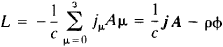where jμ is the four-current density; here j is a function of and j, where ρ is the charge density and j is the current density. Observed physical quantities, such as field strength and probabilities of electromagnetic processes, remain unchanged under a gauge transformation of the four-vector potential: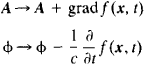where f (x,t) is an arbitrary function of the coordinates x and time t. This property, which is characteristic of the electromagnetic interaction, is called gauge invariance. One of the symmetry principles in nature (seeSYMMETRY), gauge invariance expresses in the most general form the fact of the existence of electromagnetic fields or photons and of the electromagnetic interaction. The generalization of gauge invariance to weak interactions has made it possible to formulate a unified theory of the weak and electromagnetic interactions of leptons (seeWEAK INTERACTION).

Effects of quantum electrodynamics. Effects in which a charge may be regarded as a point in an interaction with an electromagnetic field include the following: the scattering of photons by electrons, or the Compton effect; bremsstrahlung, or braking radiation; e+e or μ+ μ pair production by the Coulomb field of a nucleus; and shifts of the energy levels of atoms owing to vacuum polarization (seeVACUUM). Quantum electrodynamics, which was developed to describe atomic phenomena, turned out to be valid for distances that are considerably smaller than atomic distances. The validity of quantum electrodynamics down to distances of ~10–15 cm was determined by means of the following: studies of electron scattering by electrons and of annihilations of the type e+ + e → μ+ + μ at high energies of the colliding particles, that is, at energies of up to ~6 GeV in the center-of-mass system; studies of e+ e and μ+μ. pair production with large relative momenta of the particles and antiparticles; extremely accurate measurements of electron energy levels in atoms; and extremely accurate measurements of the anomalous magnetic moments of the electron and the muon. With a high degree of accuracy, the predictions of quantum electrodynamics agree with experimental data. For example, discrepancies between the theoretical and experimental values of the magnetic moment of the muon have not been found at the level of 10–7 percent.

A characteristic of electrodynamic processes at high energies E—that is, at Emc2. where m is the mass of the electron or muon—is that the angular distributions of the products of the processes are peaked forward of some angle. In other words, most of the outgoing particles, which may be γ, e±, or μ±, are emitted within an angle of θ ≲ mc2/E with respect to the direction of the incoming particles.

The main computational method of quantum electrodynamics is perturbation theory. Owing to the weakness of the electromagnetic interaction, the scattering matrix of processes in which electromagnetic fields participate may be expanded in a power series in the small parameter a. In calculations, only the first few terms of the series, usually not more than four terms, are considered.

In the diagrammatic technique of perturbation theory (seeFEYNMAN DIAGRAM), the simplest quantum electrodynamic process—the interaction of a photon and a structureless charged particle, that is, a charged particle represented as a point—is an integral part of any electrodynamic process. Owing to the small-ness of a, processes that include a large number of such interactions are less probable. However, such processes can be observed and are exhibited in radiative corrections, in the effects of vacuum polarization, and in multiphoton processes. In particular, vacuum polarization results in the scattering of light by light (Figure 1, a), an effect that is not found in classical electrodynamics. However, the effect is observed in the scattering of photons by the Coulomb field of a heavy nucleus (Figure 1, b).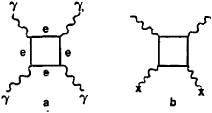Figure 1. Feynman diagrams for the scattering of light by light, γ+γ → γ + γ: (a) in quantum electrodynamics; the wavy lines represent photons, and the straight lines represent the electrons and positrons of the vacuum. The process is observed in (b) the scattering of photons by the Coulomb field of a nucleus, that is, by virtual photons; the crosses represent the Coulomb field of the nucleus.

Despite the substantial difference in the masses of the electron and the muon, no difference in the nature of the electromagnetic interaction for electrons or positrons and for muons has been observed. The lack of a difference in the interaction for electrons and muons is the basis of μ-e universality, which is yet to be explained theoretically.

Hadrons and atomic nuclei. Electromagnetic processes in which hadrons participate include photonuclear reactions that result in the production of mesons, the scattering of electrons or muons by protons or nuclei, and e+ e annihilation with the formation of hadrons. In such processes, the electromagnetic field is well studied, making the electromagnetic interaction an exceptionally effective tool for investigating the structure of hadrons and the nature of strong interactions.

As mentioned earlier, strong interactions play an important role in electromagnetic processes in which hadrons participate. For example, excited states of hadrons, or resonances, may be created by photons. Resonances are clearly exhibited in, for example, the total cross sections for the absorption of photons by protons with the formation of hadrons (Figure 2). The electromagnetic properties and electromagnetic structure of hadrons—for example, their magnetic moments, polarizability, and charge and current distributions—result from a cloud of virtual particles, mainly pions, that are emitted by the hadrons. For example, the mean-square radius of the charge distribution in the proton is determined by the size of the cloud and amounts to ~0.8 × 10–13cm (seeFORM FACTOR). Together with weak interactions, the electromagnetic interaction is responsible for differences in the masses of charged and neutral particles—for example, the proton and the neutron or charged pions (π±) and the neutral pion (π0)—in isotopic multiplets.

Because of the short-range nature of strong interactions, only the lowest multipole moments of a photon may participate in reactions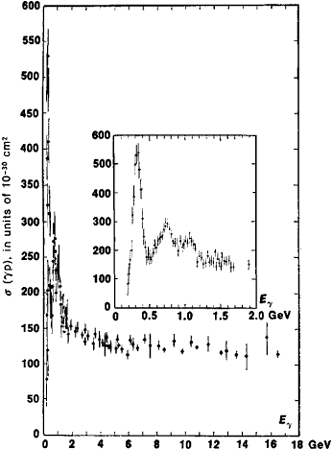Figure 2. Dependence of the total cross section σ (γp) for the absorption of photons by protons, which results in the formation of hadrons, on photon energy Eγ in the laboratory system; the maximums correspond to the creation of nucleón resonances by photons

at energies E <ℏc/R, where R is the size of a hadronic system. Consequently, the angular dependence of the differential cross sections is a smooth curve. At high energies (E > 2 GeV), the angular dependences and energy dependences of the characteristics, such as cross sections and polarizations, of electromagnetic interaction processes of hadrons and of purely hadronic processes are the same. In Figure 2, at E > 2 GeV, σ(γp) is slightly dependent on energy; such a slight energy dependence is characteristic of the total cross sections of hadronic interactions.

The similarity of the dependences of the characteristics of hadronic electromagnetic processes and purely hadronic processes underlies the vector-meson dominance model. According to the model, before a photon interacts with hadrons, it undergoes a transition to a hadronic state; that is, it changes into a vector meson, such as the ρ0, ω, or φ meson. The possibility of such a transition is clearly illustrated by the resonance energy dependence of the cross section of the process e+ + eK+ + K. The dependence is due to the conversion of a virtual photon in an intermediate state into a φ meson and the subsequent decay of the φ meson into a pair of kaons (Figure 3,b). A virtual photon is characterized by a nonzero value for the square of its four-momentum q2 = E2/c2p2 ≠ 0, where E is the photon energy and p is the photon momentum in three dimensions; for a real photon, q2 = 0. For example, for a virtual photon that is exchanged by an electron and a proton during scattering, q2 = – (4EE/c2) × sin2(θ/2), where E and E are the respective electron energies before and after scattering (for the case where E and Emc2) and θ is the scattering angle in the laboratory frame of reference, or the laboratory system.

Experiments have shown that the vector-meson dominance model is satisfactory for describing electromagnetic phenomena that involve either real photons or virtual photons with ǀq2ǀ<2 (GeV/c)2. In particular, in the cross section of the annihilation process e+ e → μ+ μ at an energy of l,019.5 MeV in the center-of-mass system, deviations from the predictions of quantum electrodynamics are observed that result from the formation of a θ meson in an intermediate state (see Figure 3,a). According to quantum electrodynamics, the process occurs by means of the conversion of an e+ e pair into a virtual photon γ and the conversion of γ into a μ+ μ pair.

However, the vector-meson dominance model does not describe the electromagnetic interaction of hadrons at large values of ǀq2ǀ, that is, at ǀq2ǀ >2 (GeV/c)2. For example, the measured cross section for the elastic scattering of electrons by protons decreases with increasing ǀ q2ǀ considerably more rapidly than is predicted by the model; the measured cross section depends on the spatial distribution of charge and current in a nucleón. On the other hand, the cross section for the highly inelastic scattering of electrons—that is, the cross section of the process e + p → e + hadrons with large transfers of energy and momentum to the hadronic system—decreases more slowly than the model predicts. In this case, as the total energy of the hadrons in the final state increases, the nature of the scattering approaches that of scattering by a particle represented as a point.

The circumstances of the latter case have led to the formulation of the parton model of hadrons. According to the model, a hadron is composed of partons, which behave as structureless particles in interactions with photons. The identification of partons with quarks is fruitful for an understanding of highly inelastic scattering.

Despite the fact that the electromagnetic interaction is the most fully studied of the known fundamental interactions, it remains a subject of active research at many scientific centers. The study of the electromagnetic interaction continues both because of the remarkable variety of microscopic and macroscopic manifestations of the interaction that are of practical value and because of the unique role of the electromagnetic field, as a well-studied subject, in the investigation of the structure of matter at extremely small distances, in obtaining information about the other known fundamental interactions, and in the discovery of new laws and symmetry principles in nature. Basic research of such types is carried out by using extremely accurate methods of atomic and nuclear spectroscopy. Such research is performed either in cosmic rays or by means of intense beams of high-energy photons, electrons, or muons produced in accelerators.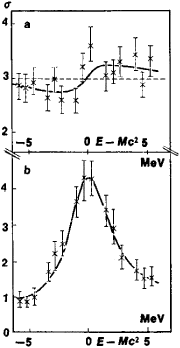Figure 3. The behavior of the cross sections σ (in arbitrary units) of the following processes near the threshold for φ meson production: (a) e+ + e → μ+ + μ and (b) e+ + e → K+ + K. The difference E — Mc2, where E is the total energy in the center-of-mass system and M is the rest mass of the φ meson (Mc2 = 1,019.5 MeV), is given on the axis of abscissas. The broken line in (a) represents the prediction of quantum electrodynamics; the solid lines represent the results of calculations that take into account the conversion of a virtual photon into a φ meson and the subsequent decay of the φ meson into a μ+μ pair through a virtual photon or into K+ + K. The experimental points were obtained on a device with colliding e+e beams.

REFERENCES

Elektromagnitnye vzaimodeistviia i struktura elementarnykh chastits. Moscow, 1969. (Translated from English.)
Akhiezer, A. I., and V. B. Berestetskii. Kvantovaia elektrodinamika, 3rd ed. Moscow, 1969.
Feld, B. Modeli elementarnykh chastits. Moscow, 1971. (Translated from English.)
Feynman, R. Vzaimodeistvie fotonov s adronami. Moscow, 1975. (Translated from English.)
Weinberg, S. “Svet kak fundamental’naia chastitsa.” Uspekhi fizicheskikh nauk, 1976, vol. 120, issue 4. (Article translated from English.)

A. I. LEBEDEV

References in periodicals archive ?
From the perspective of interactions, the electromagnetic interaction includes the electromagnetic fundamental field and adjoint quantumfield.
would correspond to weak interaction terms while the [l.sup.3] twist disclination terms would correspond to electromagnetic interaction terms.
Einstein had tried to find a mathematical treatment that could cover both gravitational and electromagnetic interactions (at a time when the strong and weak interactions had not yet been discovered) and had failed.
In the same way, the short range Yukawa potential could be regarded as a screened initially long range fictitious potential, and we consider also the electromagnetic interaction to be screened as well, because now the flux of incoming particles should be normalized for a wave packet of the relevant size rather than for a plane wave.
where [j.sup.[mu]] denotes the charge's 4-current and the last term of (3) represents the electromagnetic interaction.
Like the electromagnetic interaction, the weak interaction between quarks and leptons is understood at the fundamental level and is weak enough to probe strongly interacting systems without affecting the strong dynamics.
In understanding the basic concepts of unification of the four cosmological interactions, the cosmic radius (c/[H.sub.0]) can be considered as the infinite range of the gravitational or electromagnetic interaction. Within the Hubble volume it is noticed that: 1) Each and every point in free space is influenced by the Hubble mass.
Now let us examine the electromagnetic interaction of the three kinds of quantum mechanical particle described in the previous section.
The electromagnetic interaction is an interaction between electric charges.
It establishes magnetism as a force entirely separate from the electromagnetic interaction and converts all of classical magnetism into a quantum theory.
It was seen that a Yukawa-type potential exists at r = R for gravitational interaction as well as at r = [r.sub.e] = R/2 for electromagnetic interaction. The two related energy equations are respectively (14) and (16).

Site: Follow: Share:
Open / Close